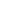# What are pips and how to calculate them correctly?

A pip is one of the basic notions on Forex. However, there are traders who do not completely understand what is a pip and cannot correctly calculate it. Let’s look at it in detail.

A pip, or, sometimes, a point, is a very small measure of change in a currency exchange rate on the forex market. Normally, on Forex, 1 pip equals 0.0001. However, there are also fractional pips. In this case a financial instrument includes the yen, one pip corresponds to 0.01 as the yen’s price is lower than other major currencies’ prices.

Let’s look at the example. In the morning, the EUR/USD pair traded at 1.3005 and in the evening the currency pair increased to 1.3085. It shows that the euro bloc currency rose by 80 pips.  Or, for instance, the USD/JPY pair was at the level of 87.75 and then declined to the score of 87.00. Consequently, the price changed by 75 pips.

On Forex a pip and a point have the same notion, but on the stock market their meanings are completely different. Thus, on the NYSE, 1 pip equals 1 cent and it is the smallest measure of change in a currency rate. At the same time, 1 point corresponds to 1 US dollar and equals 100 pips.

Now we understand the notions and difference between them. But it is not enough. It is also important to know how to convert the measure into the chosen currency. Let’s look at the US national currency. Special calculations will allow us to define the accurate amount of loss or profit. You can use the Forex calculator or make calculations yourself. At the same time, it is necessary to remember a simple formula. Using it, you can easily estimate your profits or losses when working on the currency market.

Calculation №1. This type of calculation is used for direct quotes which have the US dollar as a basic currency.  In this case, a pip equals the product of a lot size and the smallest change in price divided by the current price.

For example: the trade size of the USD/JPY financial instrument is 1 lot, the current price is 87.15 and the smallest change in the price is 0.01. As a result, a pip price is the following:

• 1 pip= 100.00 * 0.01 /87.15 = 11.47 US dollars.

Calculation №2.  Let’s consider a financial instrument with indirect quotation. The US dollar is a quoted currency. Thus, a pip corresponds to the product of a lot size and the smallest change in price.

For example: the trade size of the EUR/USD financial instrument is 1 lot and the smallest change in price is 0.0001.

1 pip for a particular currency will cost 100,000 * 0.0001 = 10 US dollars.

Calculation №3. This method is used for cross rates. These pairs do not include the US dollar. In this case, a pip equals the product of a lot size, the smallest change in price, and the main currency instrument price divided by the current currency pair price.

Let’s look at the EUR/JPY pair with a lot size of 100,000. The smallest change in price is 0.01 and the current pair’s price is 112.46. The main currency instrument is EUR/USD with the price of 1.300. Thus,1 pip equals 100,000 *0.01*1.3000*112.50 = 11.56 US dollars.Start trading with
no risks and investments
With new Start-Up Bonus of \$1000Get bonus
55%
from InstaForex
on every depositEarn up to
\$50000
for inviting friends to get StartUp Bonus from InstaForex
No investments required!